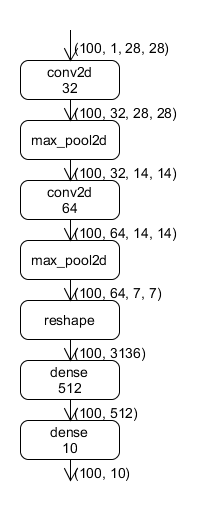# 使用OneFlow搭建神经网络¶

LeNet 是一个主要由卷积层、池化层和全连接层组成的神经网络。``````def lenet(data, train=False):
initializer = flow.truncated_normal(0.1)
conv1 = flow.layers.conv2d(
data,
32,
5,
activation=flow.nn.relu,
name="conv1",
kernel_initializer=initializer,
)
pool1 = flow.nn.max_pool2d(
conv1, ksize=2, strides=2, padding="SAME", name="pool1", data_format="NCHW"
)
conv2 = flow.layers.conv2d(
pool1,
64,
5,
activation=flow.nn.relu,
name="conv2",
kernel_initializer=initializer,
)
pool2 = flow.nn.max_pool2d(
conv2, ksize=2, strides=2, padding="SAME", name="pool2", data_format="NCHW"
)
reshape = flow.reshape(pool2, [pool2.shape, -1])
hidden = flow.layers.dense(
reshape,
512,
activation=flow.nn.relu,
kernel_initializer=initializer,
name="dense1",
)
if train:
hidden = flow.nn.dropout(hidden, rate=0.5, name="dropout")
return flow.layers.dense(hidden, 10, kernel_initializer=initializer, name="dense2")
``````

## Op 和 Layer¶

`layers.conv2d``layers.dense` 不是基本的运算单元，它们是由算子组合成的特定的运算层（Layer）。层的存在使得搭建神经网络更方便，相关接口使用请参考 oneflow.layers API

## 网络构建过程中的数据块¶

OneFlow 默认模式是静态图机制，网络的构建和运行过程其实是分开的。因此，在定义网络时，各个变量中 并没有 真实的数据，它们只是数据的占位符。真实数据的计算发生在作业函数的调用过程中。

``````print(conv1.shape, conv1.dtype)
``````

Blob 的运算符重载 `BlobDef` 中定义了运算符重载，也就是说，`BlobDef` 对象之间可以进行加减乘除等操作。

``````output = output + fc2_biases
``````

``````output = flow.broadcast_add(output, fc2_biases)
``````

## 总结¶

OneFlow 提供的算子可以参阅 API 文档中的：oneflow.nnoneflow.mathoneflow.layers 等模块。